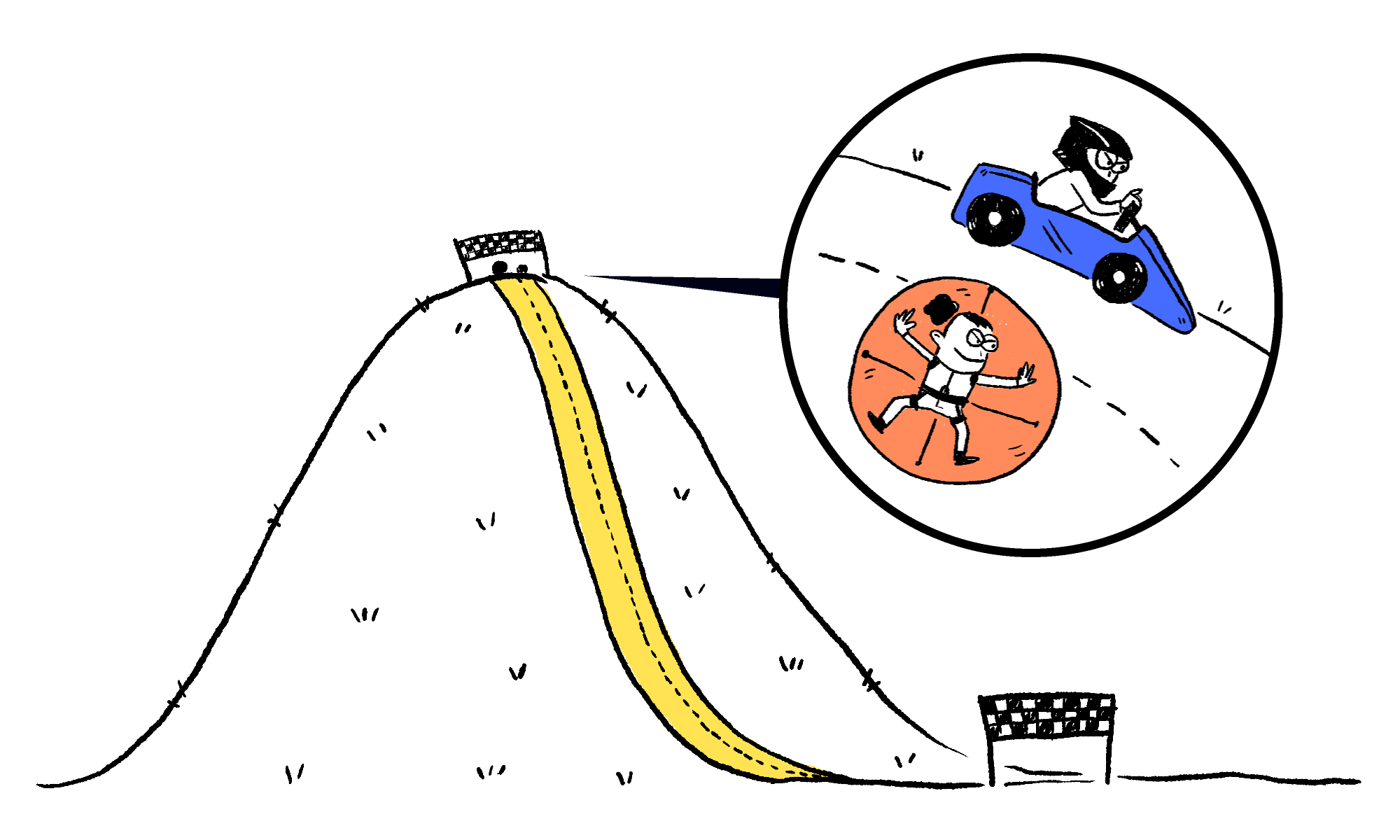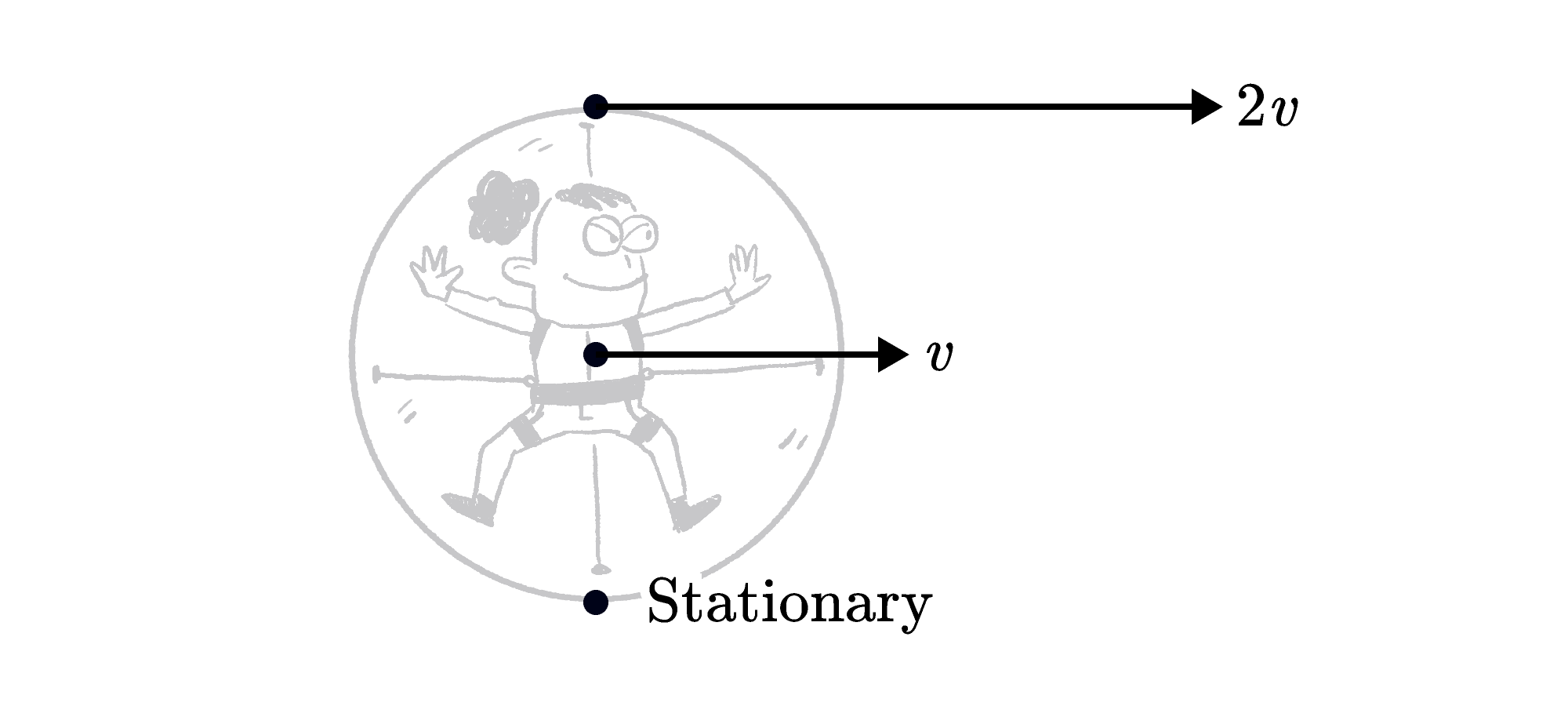Back

## Falling-Down-A-Hill Championship

Today is the inaugural world freestyle falling-down-a-hill championship: equal parts a test of engineering excellence and bravery in the face of pointless danger.Only two competitors dare take their places on the starting line at the top of a treacherous mountainside. Ride-on-Ron has brought an unpowered buggy, while Hamster-ball-Saul is fastened in place in a rigid sphere. Who will make it to the bottom of the mountain first?

The easiest way to work out how quickly something moves as it falls, slides, or rolls downhill is by thinking about energy.

As Ron and Saul lose height and pick up speed, they lose gravitational (potential) energy and gain kinetic energy. At any moment in the race, the gravitational energy lost is equal to the kinetic energy gained. This principle, known as conservation of mechanical energy, applies to rolling objects that feel very little friction.

As fate would have it, Ron and Saul (in their respective vehicles) tip the scales at the same weight, so they have identical gravitational energies on top of the mountain as the race kicks off.

Once the race starts and they have speed $v,$ a part of their initial energy has been transformed to kinetic energy: $K = \frac{1}{2} m v^2,$ where $m$ is their mass. This equation assumes the whole object is moving at a single speed.

However, when Saul (in his ball) is rolling and the ball's center is moving with speed $v,$ the part that is in contact with the ground is temporarily stationary but the top part of the rolling object is moving faster than the middle.

We can still apply the equation $K = \frac{1}{2} m v^2,$ but we have to be careful about how we handle the parts of the ball moving at different speeds. Let's imagine that we have three small masses: at the top, middle, and bottom of the rolling object.Rolling to the right with speed $v$

Each mass $m$ has a different kinetic energy when the center of the object is moving with speed $v:$

• The speed of the middle is $v,$ so its energy is just $\frac{1}{2} mv^2.$

• The speed of the bottom is $0,$ so at this instant its kinetic energy is zero as well.

• The speed of the top is double the speed of the center since it is twice as far from the point of contact. Thus, its speed is $2v$ and its kinetic energy is $\frac{1}{2} m(2v)^2,$ or just $2mv^2$.

If you average out those three energies (top, middle, and bottom bits), you get something bigger than $\frac{1}{2} mv^2$. In other words, a rolling object has more kinetic energy than a non-rotating object moving at the same speed.

In practice, we break the object’s kinetic energy into two parts:

• translational kinetic energy — the energy of the object as a whole $\big(\frac{1}{2} mv^2\big)$ for the whole object

• rotational kinetic energy — additional kinetic energy from the object’s rotation, which depends on the speed of rotation and the distribution of mass in the body.

How the energy splits depends on the exact shape of whatever's rolling. We'll leave it to you to decide whether this impacts the outcome of the race, which ends once all of the contestant's initial potential energy has transformed to kinetic energy.

# Today's Challenge

Ride-on-Ron's buggy and Hamster-ball-Saul's sphere have equal masses when Ron and Saul are riding inside. Both are powered solely by gravity and roll without resistance.The buggy, however, glides on state-of-the-art "massless" wheels, which have very little kinetic energy compared to its body.

Who will win the race to the bottom of the mountain?We are sunsetting our community features by July 2, 2021. Learn more here.
×

Problem Loading...

Note Loading...

Set Loading...